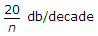# Electronics and Communication Engineering - Automatic Control Systems

Exercise : Automatic Control Systems - Section 2
1.
The first column of a Routh array is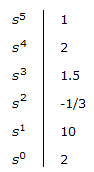How many roots of the corresponding characteristic equation are in left half s-plane?
2
3
4
5
Explanation:

Total number of roots is 5. Since two sign changes in first column, two roots are in right half plane.

Hence 3 roots in left half plane.

2.
For the given figure, time constant RC = t. Then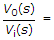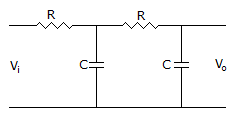t2s2 + 2ts + 1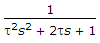ts2 + 1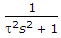Explanation: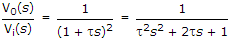.

3.
The entries in the first column of Routh array of a fourth order are 5, 2, - 0.1, 2, 1. The number of poles in the right half plane are
1
2
3
4
Explanation:

Two sign changes and hence two poles in right half plane.

4.
In Bode diagram (log magnitude plot) the factor (jω)n in the transfer function gives a line having slope